# NCERT SOLUTIONS FOR CLASS 9 SCIENCE CHAPTER 10 GRAVITATION

The National Council of Educational Research and Training (NCERT) is an autonomous body of the Indian government that formulates the curricula for schools in India that are governed by the Central Board of Secondary Education (CBSE) and certain state boards. Therefore, students who will be taking the Class 10 tests administered by various boards should consult this NCERT Syllabus in order to prepare for those examinations, which in turn will assist those students get a passing score.

When working through the exercises in the NCERT textbook, if you run into any type of difficulty or uncertainty, you may use the swc NCERT Solutions for class 9 as a point of reference. While you are reading the theory form textbook, it is imperative that you always have notes prepared. You should make an effort to understand things from the very beginning so that you may create a solid foundation in the topic. Use the NCERT as your parent book to ensure that you have a strong foundation. After you have finished reading the theoretical section of the textbook, you should go to additional reference books.

## NCERT SOLUTIONS FOR CLASS 9 SCIENCE CHAPTER 10 GRAVITATION – Exercises

Question 1. State the universal law of gravitation.
Solution : According to Newton’s universal law of gravitation :
Every mass in this universe attracts every other mass with a force which is directly proportional to the product of two masses and inversely proportional to the square of the distance between them.

Question 2. Write the formula to find the magnitude of the gravitational force between the earth and an object on the surface of the earth.
Solution : The formula to find the magnitude of the gravitational force between the earth and an object on the surface of the earth is given below:

F = magnitude of gravitational force

G = Universal gravitation constant

M = mass of earth

m = mass of object

d = distance of object from the centre of earth

Question 3. What do you mean by free fall?
Solution : It is the object falling towards earth under the influence of attraction force of earth or gravity.

Question 4. What do you mean by acceleration due to gravity?
Solution : During free fall any object that has mass experiences force towards centre of earth and hence an acceleration works as well. “acceleration experienced by an object in its free fall is called acceleration due to gravity.” It is denoted by g.

Question 5. What are the differences between the mass of an object and its weight?
Solution :

Question 6. Why is the weight of an object on the moon 1/6 th its weight on the earth?
Solution : Mass of object remains the same whether on earth or moon but the value of acceleration on moon is 1/6th of the value of acceleration on earth. Because of this weight of an object on moon is 1/6th its weight on the earth.

Question 7. Why is it difficult to hold a school bag having a strap made of a thin and strong string?
Solution : It is difficult to hold a school bag having a strap made of a thin and strong string because a bag of that kind will make its weight fall over a small area of the shoulder and produce a greater pressure that makes holding the bag difficult and painful.

Question 8. What do you mean by buoyancy?
Solution : It is the upward force experienced by an object when it is immersed into a fluid.

Question 9. Why does an object float or sink when placed on the surface of water?
Solution : As an object comes in contact with the surface of a fluid it experiences two types of forces: gravitational force or gravity that pulls the object in downward direction and the second force is the force of buoyancy that pushes the object in upward direction.
It is these two forces that are responsible for an object to float or sink
i.e. if gravity >buoyancy then object sinks
if gravity <buoyancy then object floats

Question 10. How does the force of gravitation between two objects change when the distance between them is reduced to half?
Solution : The force of gravitation between two objects is inversely proportional to the square of the distance between them therefore the gravity will become four times if distance between them is reduced to half.

Question 11. Gravitational force acts on all objects in proportion to their masses. Why then, a heavy object does not fall faster than a light object?
Solution : In free fall of objects the acceleration in velocity due to gravity is independent of mass of those objects hence a heavy object does not fall faster than a light object.

Question 12. What is the magnitude of the gravitational force between the earth and a 1 kg object on its surface? (Mass of the earth is 6 x 1024 kg and radius of the earth is 6.4 x 106 m.)
Solution :

= 9.81 N

Question 13. The earth and the moon are attracted to each other by gravitational force. Does the earth attract the moon with a force that is greater or smaller or the same as the force with which the moon attracts the earth? Why?
Solution : According to the universal law of gravitation, two objects attract each other with equal force, but in opposite directions. The Earth attracts the moon with an equal force with which the moon attracts the earth.

Question 14. If the moon attracts the earth, why does the earth not move towards the moon?
Solution : Earth does not move towards moon because mass of moon is very small as compared to that of earth.

Question 15. What happens to the force between two objects, if
(i) the mass of one object is doubled?
(ii) the distance between the objects is doubled and tripled?
(iii) the masses of both objects are doubled?
Solution :

(i) the force between two objects will be doubled.

(ii) the force between two objects will become 1/4th and 1/9th of the present force.

(iii) the force between two objects will become four times the present force.

Question 16. What is the importance of universal law of gravitation?
Solution : The universal law of gravitation is important due to the following:

i) this law explains well the force that binds us to earth.

ii) this law describes the motion of planets around the sun.

iii) this law justifies the tide formation on earth due to moon and sun.

iv) this law gives reason for movement of moon around earth.

Question 17. What is the acceleration of free fall?
Solution :
The acceleration of free fall is  g = 9.8 m/s2(on earth)

Question 18. What do we call the gravitational force between the earth and an object?
Solution : Weight

Question 19. Amit buys few grams of gold at the poles as per the instruction of one of his friends. He hands over the same when he meets him at the equator. Will the friend agree with the weight of gold bought? If not, why? [Hint: The value of g is greater at the poles than at the equator.]
Solution :
Since W = m x g and given in the Question that value of g is greater at the poles than at the equator, hence weight of same amount of gold will be lesser at equator than it was on the poles. Therefore, the friend will not agree with the weight of gold bought.

Question 20. Why will a sheet of paper fall slower than one that is crumpled into a ball?
Solution : A greater surface area offers greater resistance and buoyancy same is true in the case of a sheet of paper that has larger surface area as compared to paper crumpled into a ball. So sheet of paper falls slower.12. Gravitational force on the surface of the moon is only 1/6 as strong as gravitational force on the earth. What is the weight in newtons of a 10 kg object on the moon and on the earth?

Question 21. Gravitational force on the surface of the moon is only 1/6 as strong as gravitational force on the earth. What is the weight in newtons of a 10 kg object on the moon and on the earth?
Solution :

value of gravity on earth g = 9.8 m/s2

value of gravity on moon = 1/6th of earth = 9.8/6 = 1.63 m/s2

weight of object on moon = m x 1.63 = 10 x 1.63 = 16.3 N

weight of object on earth = m x 9.8 = 10 x 9.8= 98 N

Question 22. A ball is thrown vertically upwards with a velocity of 49 m/s. Calculate
(i) the maximum height to which it rises,
(ii) the total time it takes to return to the surface of the earth.
Solution :
(i) v = u + gt

0 = 49 + (-9.8) x t

9.8t = 49

t = 49/9.8 = 5 s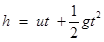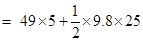= 245 – 122.5 = 122.5

(ii) total time taken to return = 5 + 5 = 10 s

Question 23. A stone is released from the top of a tower of height 19.6 m. Calculate its final velocity.
Solution :

19.6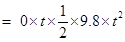4.9 t2 = 19.6

= t2 = 19.6/4.9= 4

t = 2

since v= u + at

= 0 + 9.8 x 2= 19.6 m/s

Question 24. A stone is thrown vertically upward with an initial velocity of 40 m/s. Taking g=10 m/s2, find the maximum height reached by the stone. What is the net displacement and the total distance covered by the stone?
Solution :
Initial velocity of stone (u) = 40 m/s

at maximum height stone will be at rest so v= 0

v = u + gt

= 0 = 40 + (-10) x t

10t = 40

t = 40/10 = 4 s

distance covered /maximum height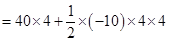= 160 – 80 = 80 m

net displacement of stone = 0(thrown upwards then falls back to same place)

total distance covered by the stone = 80 + 80 = 160 m

Question 25. Calculate the force of gravitation between the earth and the Sun, given that the mass of the earth = 6 x 1024 kg and of the Sun= 2x 1030 kg. The average distance between the two is 1.5 x 1011 m.
Solution :

= 35.73 x 1021

Question 26. A stone is allowed to fall from the top of a tower 100 m high and at the same time another stone is projected vertically upwards from the ground with a velocity of 25 m/s. Calculate when and where the two stones will meet.
Solution :
Suppose both the stones will meet after t seconds.

= 5t2

= 25t – 5t2

h + h’ = 100 m

5t2 + 25t – 5t2  = 100

25t = 100

t = 4 s

h = 5t2 = 5 x 4 x 4
= 80 m

Therefore, the two stones will meet after 4 seconds when the falling stone would have covered a height of 80 m.

Question 27. A ball thrown up vertically returns to the thrower after 6 s. Find
(a) the velocity with which it was thrown up,
(b) the maximum height it reaches, and
(c) its position after 4 s.
Solution :
(a)time taken by ball to reach maximum height(t) = 6/2 = 3 s

v = u + gt

0 = u + (-9.8) x 3

u = 29.4 m/s (the velocity with which it was thrown up)

(b) the maximum height it reaches: therefore= 88.2 – 44.1

= 44.1 m

(c) its position after 4 s will be:

Since in first 3 s it will reach the maximum height and in next 1 s it will start a free fall so, u = 0, t =1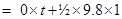= 4.9 m

therefore, after 4s the position of ball = 44.1 – 4.9 = 39.2 m

Question 28. In what direction does the buoyant force on an object immersed in a liquid act?
Solution : In the upward direction only.

Question 29. Why does a block of plastic released under water come up to the surface of water?
Solution : Since of plastic has density very less as compared to water i.e. weight of plastic is less than the buoyant force experienced by it therefore a block of plastic released under water come up to the surface of water/floats.

Question 30. The volume of 50 g of a substance is 20 cm3. If the density of water is 1 g cm–3, will the substance float or sink?
Solution :
Density of that substance (d) = mass/volume = 50/20 = 2.5 g / cm3

since the density of substance (2.5) is greater than the density of water (1) therefore it will sink.

Question 31. The volume of a 500 g sealed packet is 350 cm 3. Will the packet float or sink in water if the density of water is 1 g cm–3? What will be the mass of the water displaced by this packet?
Solution :
Density of the packet = mass/volume = 500/350 = 1.428 g / cm3

Since the density of packet is more than density of water so it will sink. And packet will displace water equal to its volume :

volume of water displaced by packet =350 cm3(volume of packet)

mass of water displaced = volume of water displaced x density of water

= 350 x 1 = 350 g

## Conclusions for NCERT SOLUTIONS FOR CLASS 9 SCIENCE CHAPTER 10 GRAVITATION

SWC academic staff has developed NCERT answers for this chapter of the ninth grade science curriculum. We have solutions prepared for all the ncert questions of this chapter. The answers, broken down into steps, to all of the questions included in the NCERT textbook’s chapter are provided here. Read this chapter on theory. Be certain that you have read the theory section of this chapter of the NCERT textbook and that you have learnt the formulas for the chapter that you are studying.